# RD Sharma Solutions for Class 9 Maths Chapter 2 Exponents of Real Numbers Exercise 2.1

RD Sharma Class 9 Mathematics Chapter 2 Exercise 2.1 Exponents of Real Numbers is provided here. Class 9 chapter 2 solutions are solved by experts in accordance with CBSE syllabus for 9th standard. This study material contains RD Sharma solutions class 9 exercise 2.1.

## Download PDF of RD Sharma Solutions for Class 9 Maths Chapter 2 Exponents of Real Numbers Exercise 2.1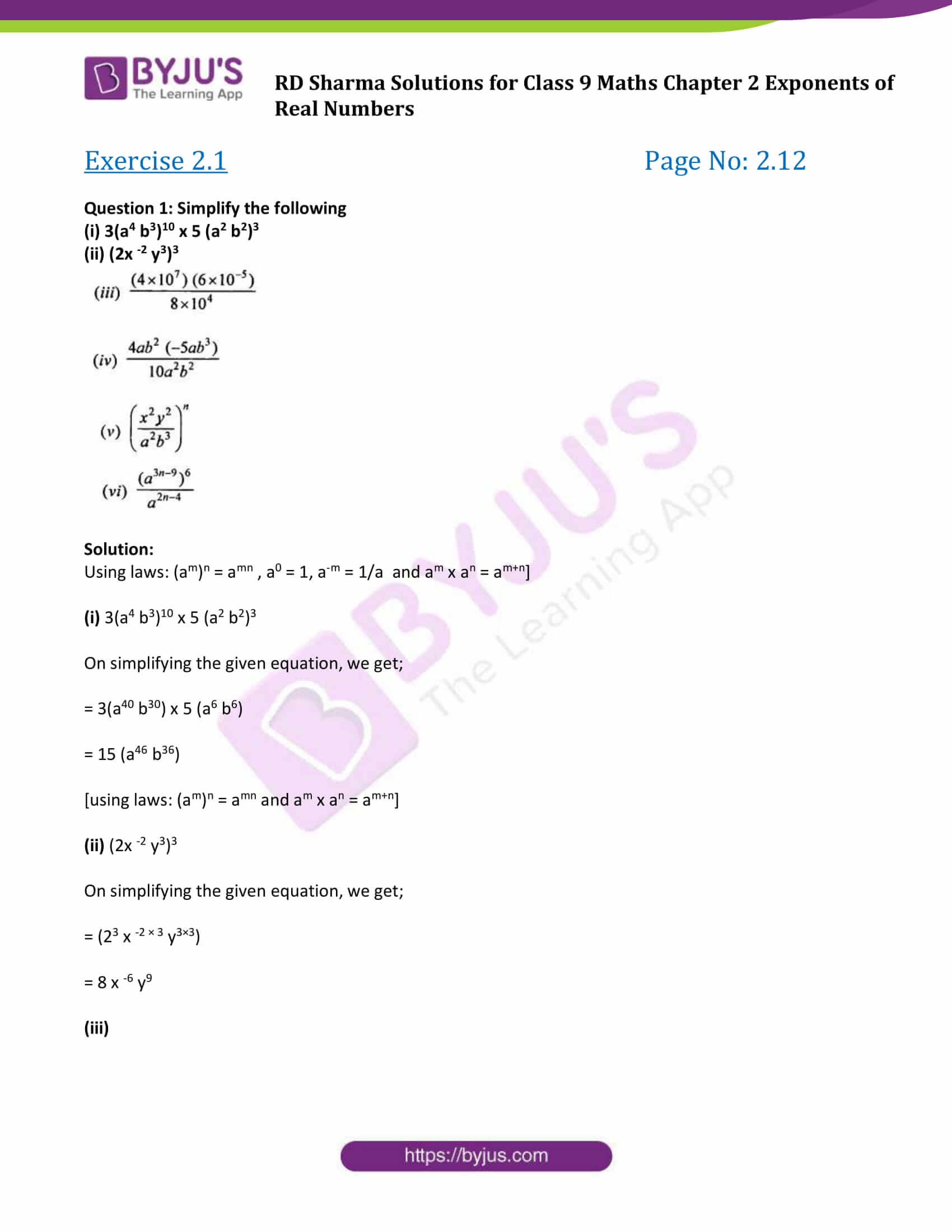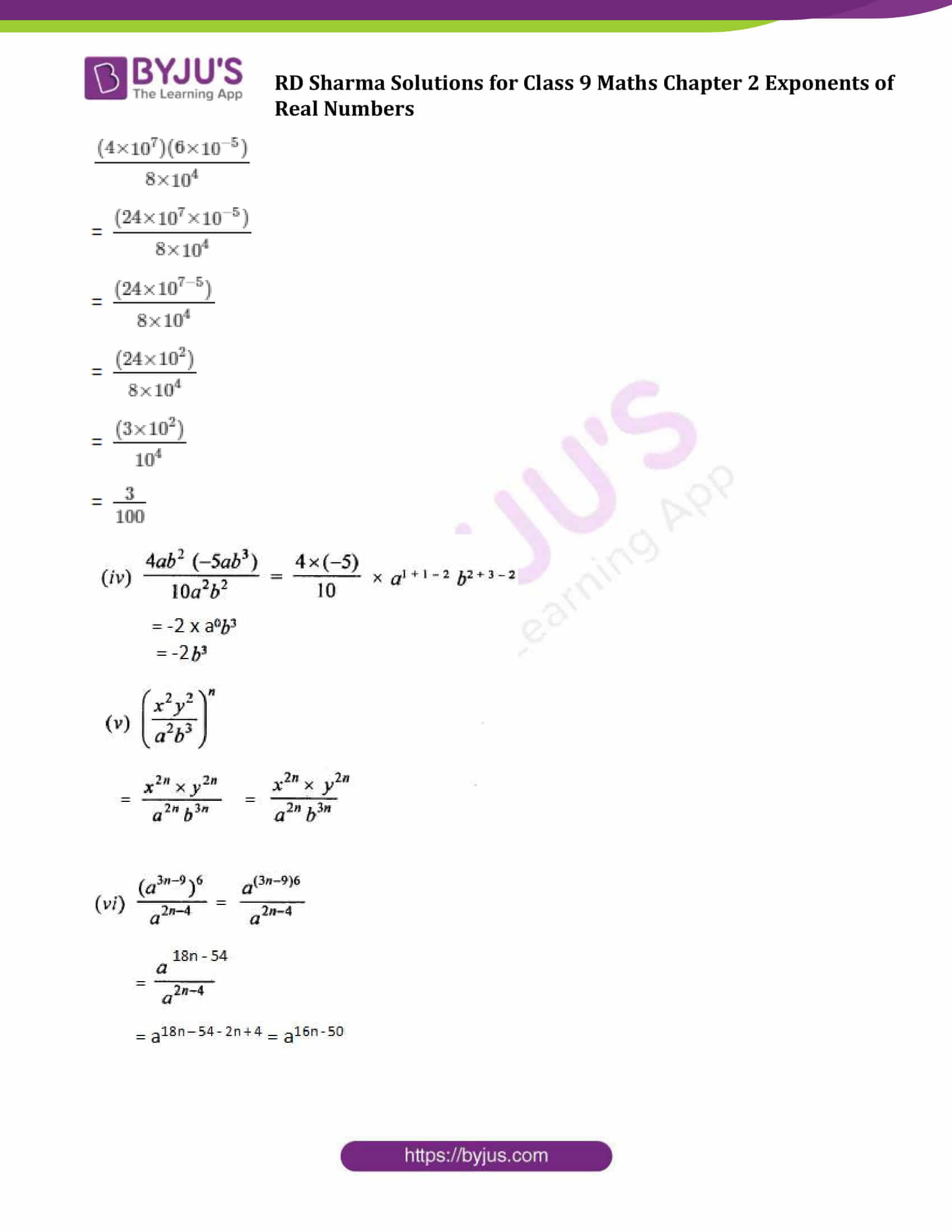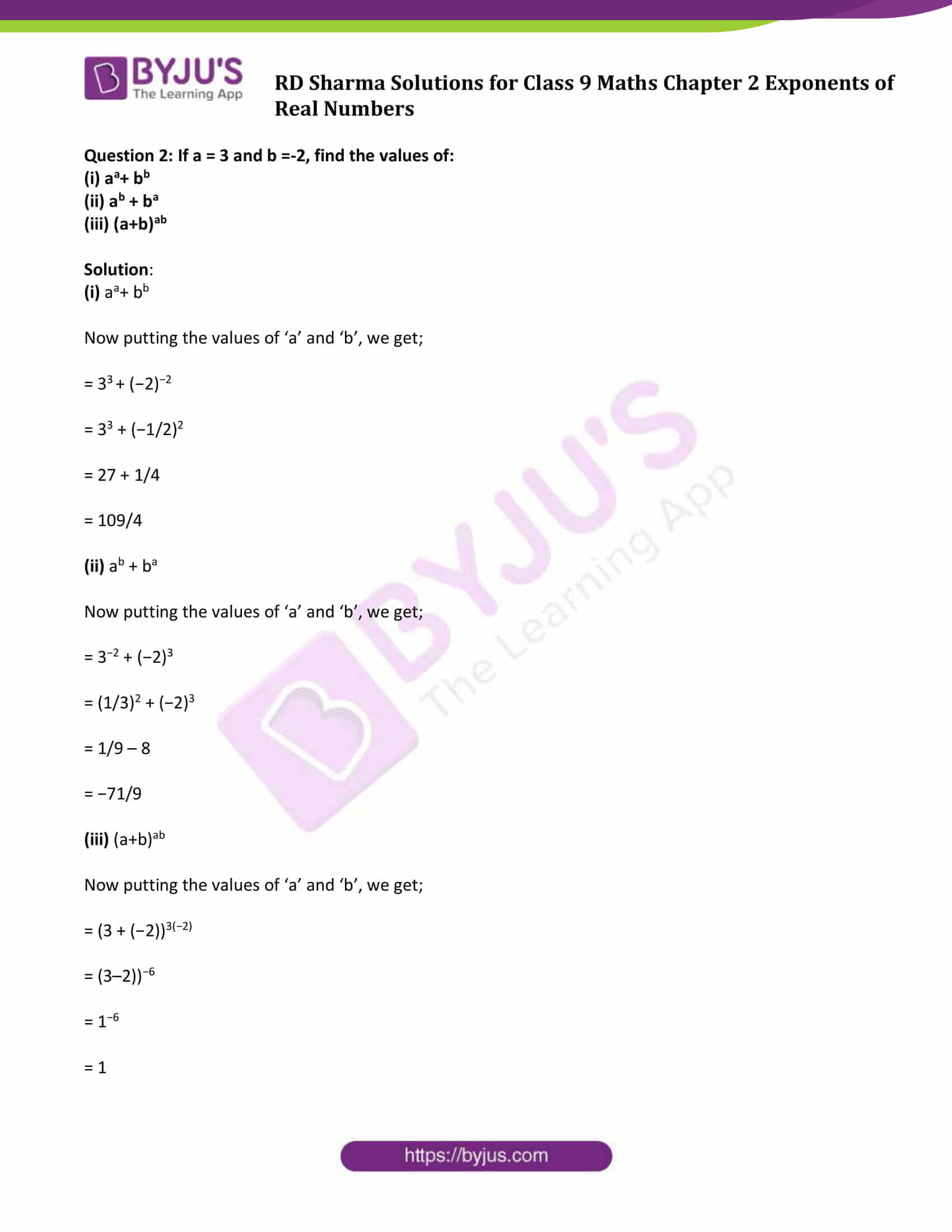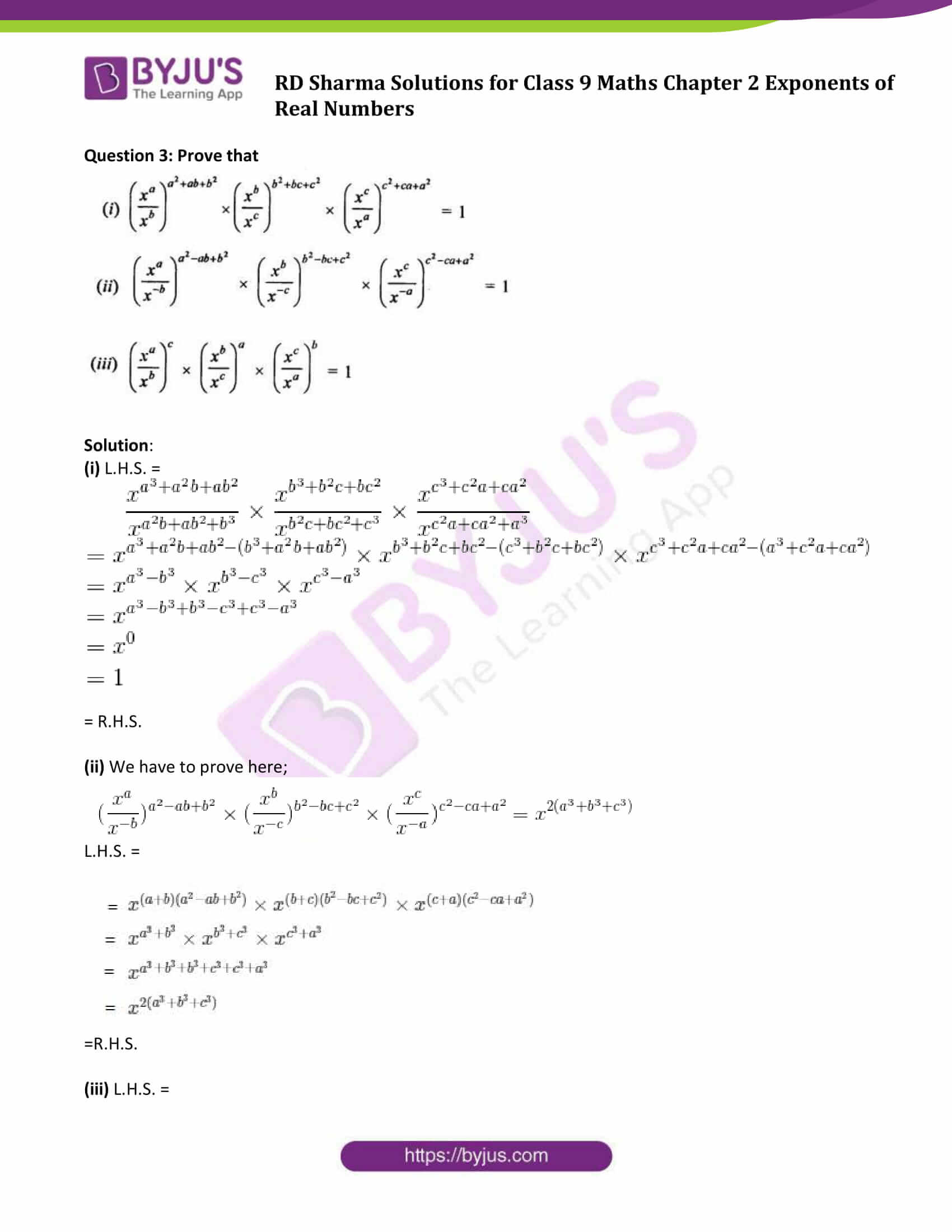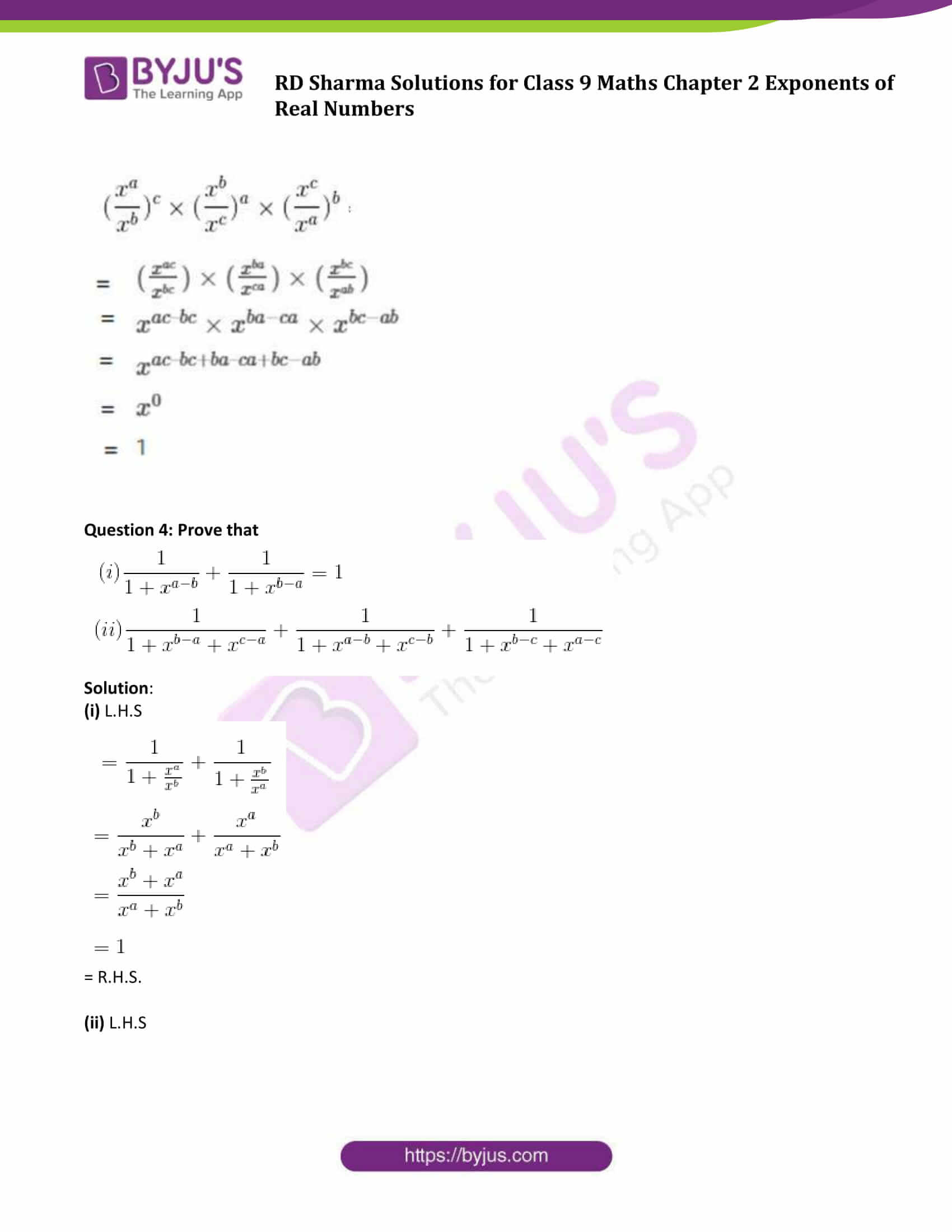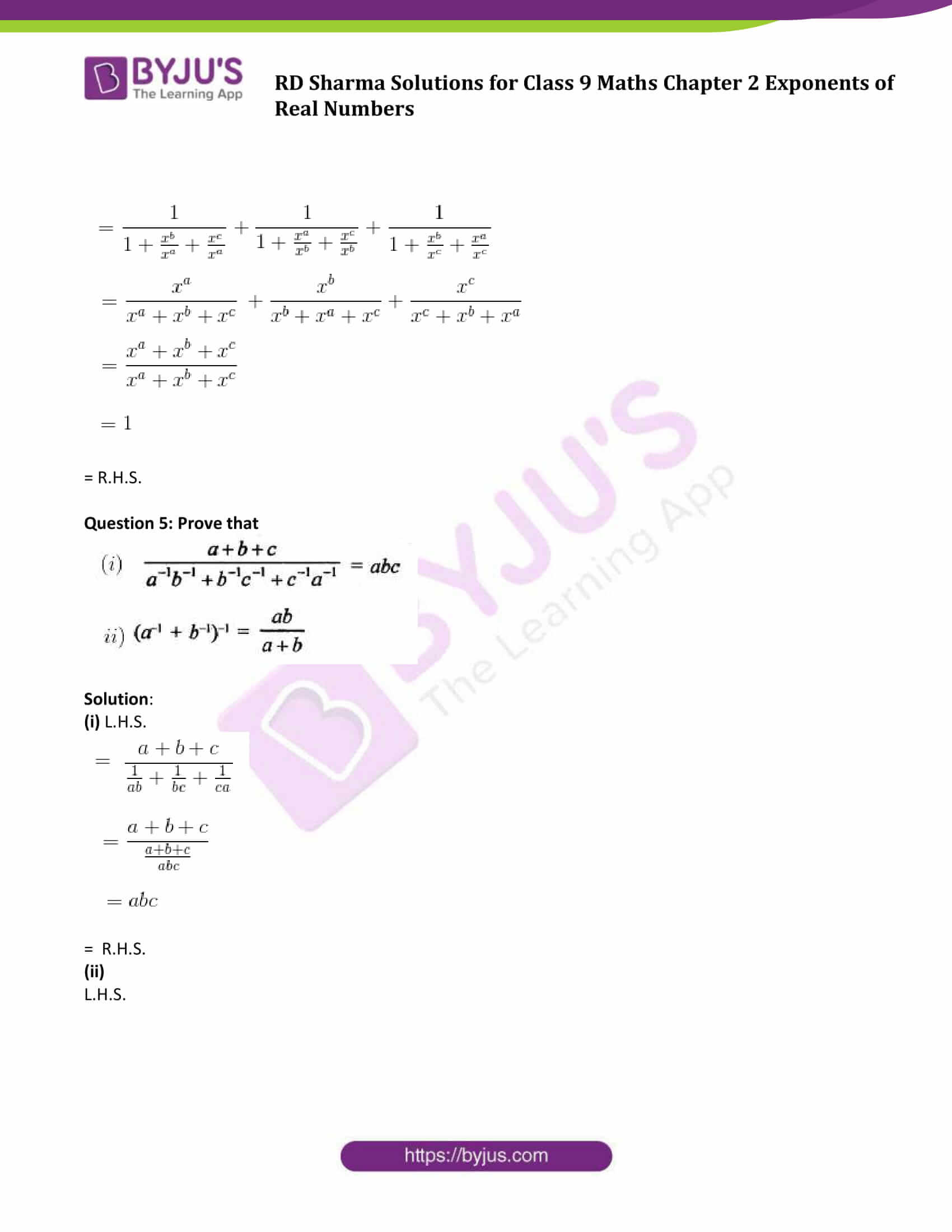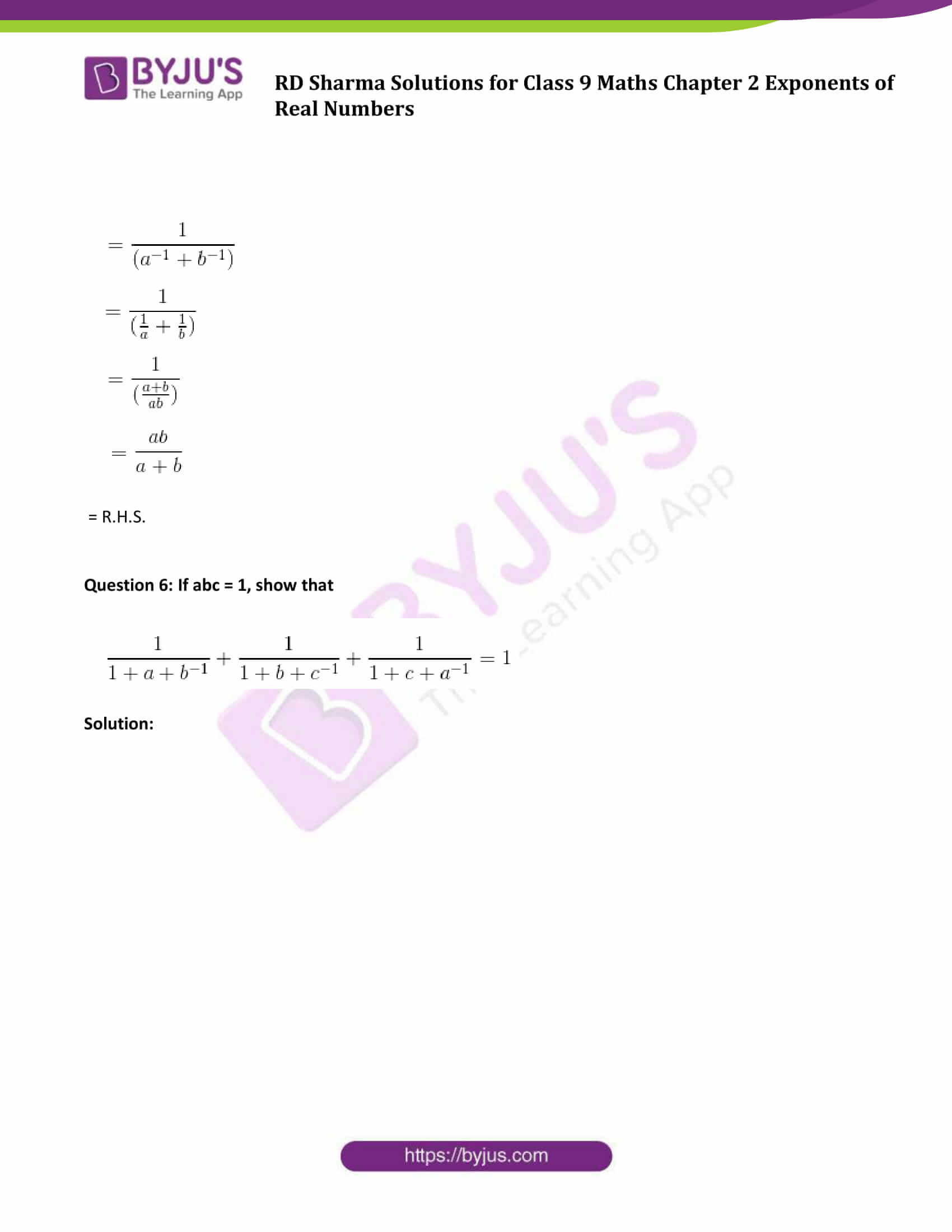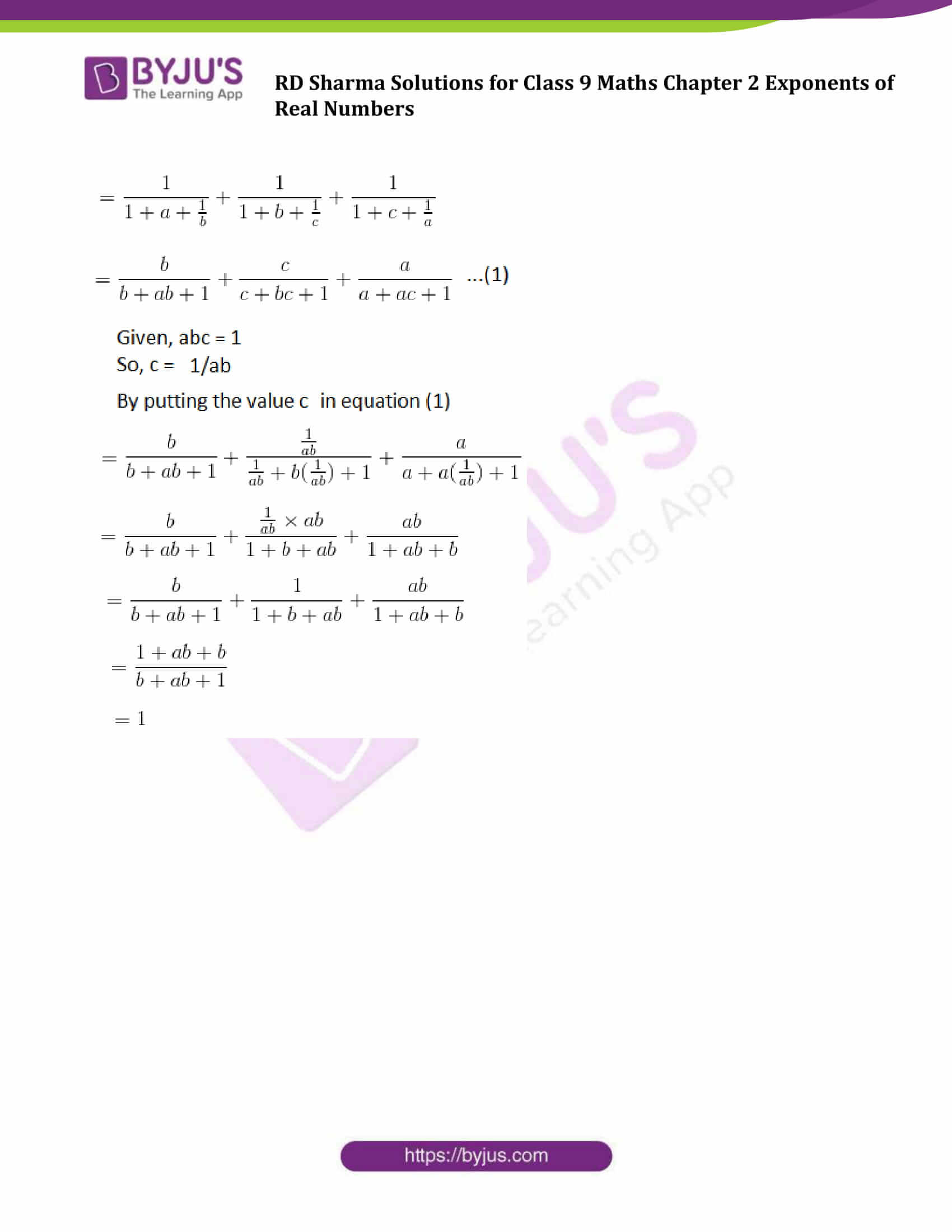### Access Answers to Maths RD Sharma Solutions for Class 9 Chapter 2 Exponents of Real Numbers Exercise 2.1 Page number 2.12

Question 1: Simplify the following

(i) 3(a4 b3)10 x 5 (a2 b2)3

(ii) (2x -2 y3)3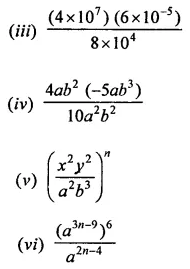Solution:

Using laws: (am)n = amn , a0 = 1, a-m = 1/a and am x an = am+n]

(i) 3(a4 b3)10 x 5 (a2 b2)3

On simplifying the given equation, we get;

= 3(a40 b30) x 5 (a6 b6)

= 15 (a46 b36)

[using laws: (am)n = amn and am x an = am+n]

(ii) (2x -2 y3)3

On simplifying the given equation, we get;

= (23 x -2 × 3 y3×3)

= 8 x -6 y9

(iii)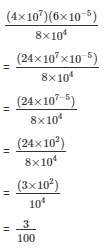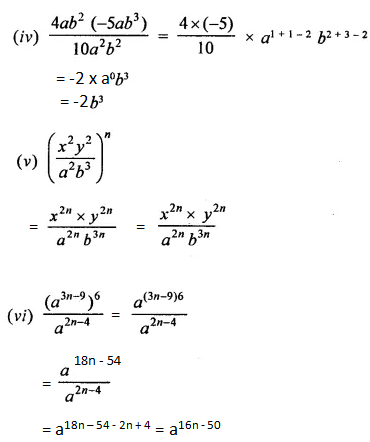Question 2: If a = 3 and b =-2, find the values of:

(i) aa+ bb

(ii) ab + ba

(iii) (a+b)ab

Solution:

(i) aa+ bb

Now putting the values of ‘a’ and ‘b’, we get;

= 33 + (−2)−2

= 33 + (−1/2)2

= 27 + 1/4

= 109/4

(ii) ab + ba

Now putting the values of ‘a’ and ‘b’, we get;

= 3−2 + (−2)3

= (1/3)2 + (−2)3

= 1/9 – 8

= −71/9

(iii) (a+b)ab

Now putting the values of ‘a’ and ‘b’, we get;

= (3 + (−2))3(−2)

= (3–2))−6

= 1−6

= 1

Question 3: Prove that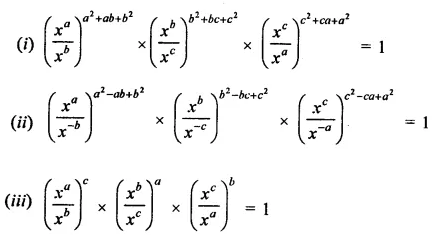Solution:

(i) L.H.S. =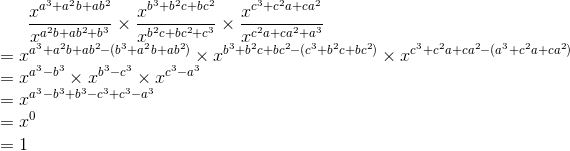= R.H.S.

(ii) We have to prove here;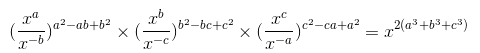L.H.S. =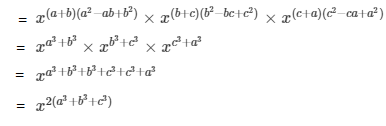=R.H.S.

(iii) L.H.S. =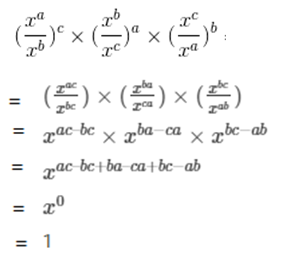Question 4: Prove that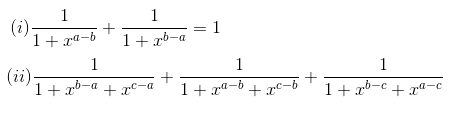Solution:

(i) L.H.S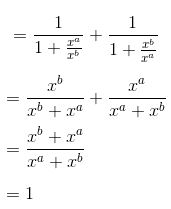= R.H.S.

(ii) L.H.S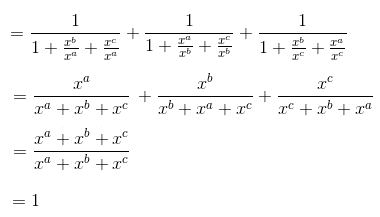= R.H.S.

Question 5: Prove that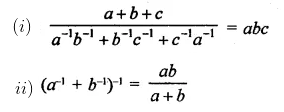Solution:

(i) L.H.S.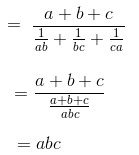= R.H.S.

(ii)

L.H.S.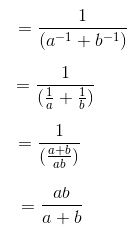= R.H.S.

Question 6: If abc = 1, show that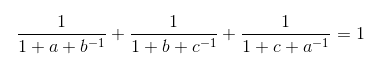Solution: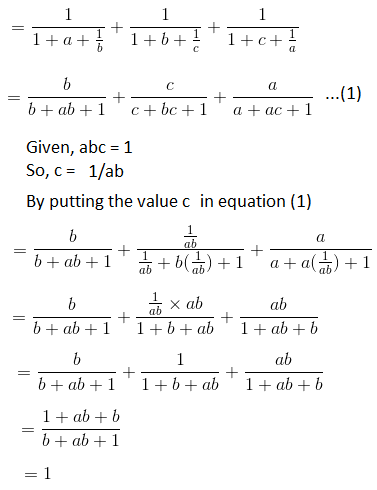## RD Sharma Solutions for Class 9 Maths Chapter 2 Exponents of Real Numbers Exercise 2.1

RD Sharma Solutions Class 9 Maths Chapter 2 Exponents of Real Numbers Exercise 2.1 is based on the following topics and subtopics:

• Exponents of Real Numbers Introduction
• Integral exponents of a real number
• Positive integral power and negative integral power
• Laws of integral exponents

First law: If a is any real number and m, n are positive integers, then am x an = a(mn)

Second Law: If a is non-zero real number and m, n are positive integers, then am / an = a(m-n)

Third law: If a is any real number and m, n are positive integers, then (am)n = a(mn) = (an)m

Fourth Law: If a and b are real numbers and m, n are positive integers then (ab)n = an bn and (a/b)n = an/bn, where b is not equal to zero.